There are some circumstances in which you might wish to display one or more questions to your respondents at random. For this you can use Routing to show particular questions to the respondent based on the value of a variable or a derived variable.

This worksheet explains how to create a derived variable based on the time a respondent accesses the survey, which is then used to select a route the respondent takes through the survey. This is not true randomness, as it will be evenly distributed across the number of routes, but the route each respondent is assigned is essentially random.

### Background

To set respondents randomly on different routes through the survey you need to firstly set up the questions for the possible routes through the survey. In this example we are assuming that there are three routes through the survey. You then have to create a derived variable that calculates the number of seconds in the minute that the respondent accessed the survey and in this example it will be if the respondent accesses the survey between 1 and 19 seconds in the minute, 20 and 39 seconds in the minute and finally 40 and 60 seconds in the minute. This will give you three codes which will be assigned as each respondent accesses the survey. The codes in the derived variable are then used in Routing Rules to route the respondents through the survey in one of the three possible routes.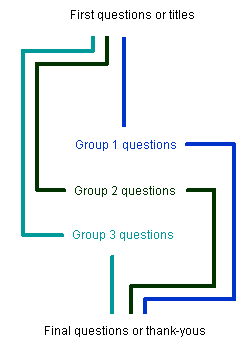Since this technique uses derived variables, it will only work on Snap WebHost and mobile interviewer surveys.

Snap can automatically record the time a respondent starts the survey in the id.start paradata variable. The Seconds can be extracted from this time and used in the derived variable to assign a code. As one minute has sixty seconds which can be broken down into a number of factors, this is a convenient way of splitting the codes into a number of groups.

### Summary of steps

This worksheet assumes that you have already created all the questions in your survey.

1. Open the survey in Questionnaire mode.
2. Click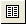to open the survey properties.
3. Select Paradata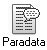in the lefthand column.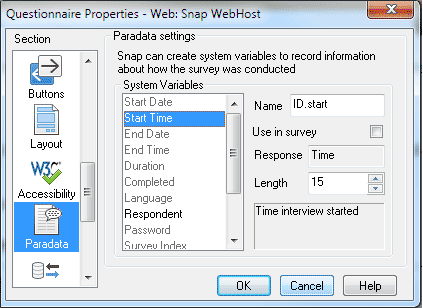4. Select the Start Time system variable and check the Use in survey box. Click [OK].
5. Save the changes to the Questionnaire by clicking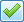.

### Step 2: Setting up the derived variable

1. Click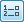to open the Variables window.
2. Click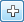to create a new variable.
3. Set the Response type to Single.
4. Add the values for the three codes.
Any respondent accessing the survey between 0 and 19 seconds in the minute will be in Route 1 and the value will be id.start second<20.
Any respondent accessing the survey between 0 and 39 seconds in the minute will be in Route 2 and the value will be id.start second<40.
Any respondent accessing the survey that has not been counted in the other 2 codes will be in Route 3 and the value will be true.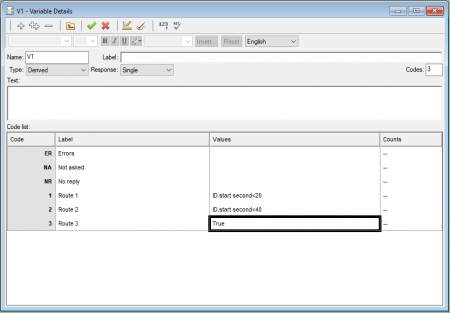This uses the second time function to extract the seconds from the id.start paradata variable. The first code is selected if the respondent started the survey at some time plus 0 – 19 seconds. If that isn’t true, the second code tests if they started the survey at some time plus 0 – 39 seconds (as this is a single response question this is equivalent to 20 – 39 seconds) and the third code is chosen otherwise.

### Step 3: Setting up your survey routing

Depending on the number of questions that you wish to display randomly you may choose different methods of routing. In this worksheet example it will be using Goto After Question routing to direct respondents to different routes. It also assumes the 3 sets of random questions in each route will be as follows:

Route 1 is questions Q5 to Q10
Route 2 is questions Q11 to Q15
Route 3 is questions Q16 to Q20

1. Select the question or text immediately before the random question sets appear.
2. Right-click and select Routing Rules from the context menu.3. Click [Add] to create a new rule.
4. Select Goto After Question from the Type dropdown list and click [OK].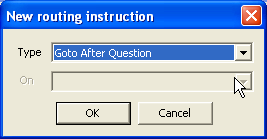5. Select the first question of Route 2 (Q11) in the Goto dropdown list, and set the If condition of `v1==2` (where v1 is the derived variable that you just created from the start time).
6. Click [Add] and set up a second Goto After Question which selects the first question of Route 3 (Q16) in the Goto dropdown list and an If condition of v1==3. Click [OK] to close the routing rules dialog.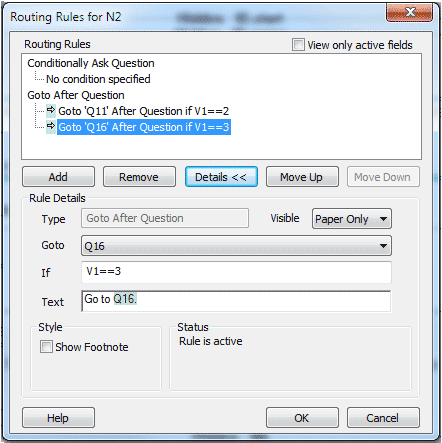This should cause respondents assigned to Route 1 to go to the first set of random questions, respondents assigned to Route 2 to go to the second set of random questions, and respondents assigned to Route 3 to go to the third set of random questions.
7. Now go to the end of the first set of random questions and add another Goto After Question that jumps respondents to the question directly after all the 3 sets of random questions. (You do not need an If clause). Click [OK].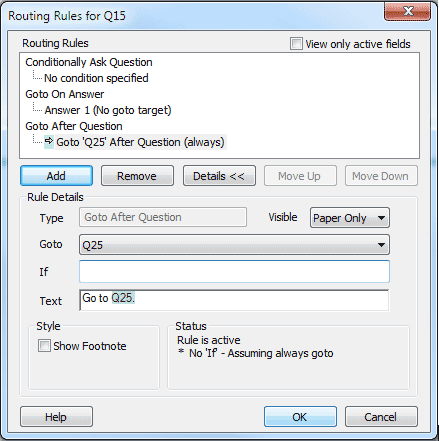8. Repeat this at the end of the second set of random questions. (The last random set of questions will just drop into the next question automatically.)

### Publish and test your survey

Publish your survey in preview mode and check that the routing is working correctly. Since you should be randomly assigned to a route according to your start time, you may need to run the survey several times in quick succession in order to find the different routes.

If there is a topic you would like a worksheet on, email to snapideas@snapsurveys.com